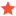Create targets based on tool positionKees12ProgrammingJunior MemberPosts: 5 Threads: 4 Joined: Mar 2023 Reputation: 0 05-10-2023, 09:50 AM Hello, Im trying to make a program that can automatically add targets to RoboDK based on the tool position. I am stuck in translating the rotation into my targets. Code:```ref_frame = kuka.Pose() pos_ref = ref_frame.Pos() #targets = np.array([*3]*len(xcoordinates)) targets = [] target = np.array([]) for i in range(0, len(xcoordinates)):    target = transl(ycoordinates[i], xcoordinates[i], 0)*pos_ref    targets.append(target) prog = RDK.AddProgram('ForwardAuto') prog.setTool(tool) prog.setRounding(10) prog.setSpeed(speed_linear=speed, accel_linear=accel) for i in range(0, len(targets)):    ti = RDK.AddTarget('AutoTarget %i' % (i + 1))    pose_i = ref_frame    pose_i.setPos(targets[i])    ti.setPose(pose_i)    ti.setAsCartesianTarget()    prog.MoveL(ti)```The list of coordinates is very simple, its split in 2 seperate arrays. the list is increasing and supposed to be from left to right. translated for the first position to be 0,0 example: xcoordinates = [0, 10, 30, 40, 50], ycoordinates = [0, 1, 3, 1, -1] This works fine, but when i rotate the robot my targets are placed in the wrong direction. so i want to either correctly translate the targets to just follow the tool coordinate system, i think this is the correct approach. Or i want to be able to translate the targets to be -1 when the base axis is over a treshhold. a picture to show the setup in roboDK is attached You can see the positive direction of the kuka frame is to the left, but i want to add points going to the right. You can also see the many points created going from the start towards the left, instead of to the right to finish. Find ReplyAlbertModeratorPosts: 1,925 Threads: 1 Joined: Apr 2018 Reputation: 101 05-10-2023, 10:01 AM If you want to make movements relative to the current robot pose you should replace this line: Code:`target = transl(ycoordinates[i], xcoordinates[i], 0)*pos_ref` By this one: Code:`target = transl(ycoordinates[i], xcoordinates[i], 0)*ref_frame` The first one translates a point while the second one translates the pose. Find ReplyKees12ProgrammingJunior MemberPosts: 5 Threads: 4 Joined: Mar 2023 Reputation: 0 05-10-2023, 11:25 AM When i replace that line, i get an error message. self[0, 3] = newpos ~~~~^^^^^^ raise Exception(MatrixError, "Submatrix indices does not match the new matrix sizes", itmsz, "x", itmsz, "<-", newm, "x", newn) Exception: (, 'Submatrix indices does not match the new matrix sizes', 1, 'x', 4, '<-', range(0, 1), 'x', range(3, 4)) Find Reply